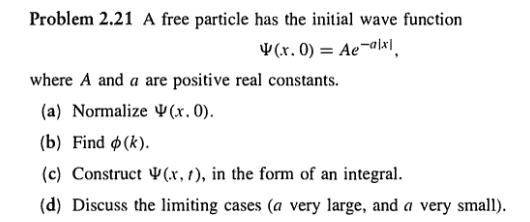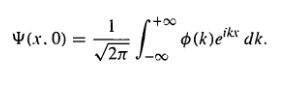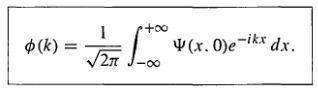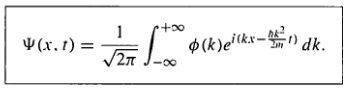# Free particle wave function behavior

## Homework Statement

Griffiths Intro Quantum Mechanics free particle question.
Normalize wave function, find Phi(k), Psi(x,t), and comment on its behavior for small and large a.
The wave function given is Ae-a|x|

## The Attempt at a Solution

I found the correct Phi(k), but for the small a vs large a, I don't understand why Phi(k) corresponds to momentum. I see that there is a small spread with a sharper peak in Psi with a flatter, more spread out Phi, and a flatter more spread out Psi with a sharper, smaller spread Phi, but I don't see how this is an example of the uncertainty principle. I don't see from reading Griffiths how Phi is any indication of momentum.[/B]

BvU
Homework Helper
Bookshop's closed at this time. Could you render the problem statement explicitly, so I can see a k too ? Or show what you have worked out so far ?

Eager to help, but without means,
BvU

Yeah I can do that in a few hours. I'm not sure how to use latex so I'll have to look that up since I don't see a way to graphically insert symbols.

In the meantime, this is the text in the book the problem refers to. It's from an older edition on my phone which doesn't have the practice problem. But I'm trying to understand how the Phi(k) corresponds to momentum. The book talking about their behaviors as an example of the uncertainty principle. I understand the behaviors, but I don't see how that integral that gives you Phi is momentum.

Here is the problem, and how Psi(x,0), Psi(x,t), and Phi(k) are constructedNow I have found parts A-C fine, with $$\Psi(x,0)$$ having a normalization constant of $$\sqrt{\frac{a}{2\pi}} \\ \phi(k) = \frac{2a}{k^2+a^2}\sqrt{\frac{a}{2\pi}}$$

For the last part, I understand how for small a Psi has a sharper peak and smaller spread, while phi is broader with a larger spread, and I see that for large a Psi is broad with a larger spread while Psi has a sharper peak, but I am failing to understand how this illustrates the uncertainty principle since I don't see how phi represents momentum. I thought phi (with the 1 over root 2 pi) would be the coefficients of the individual solutions, allowing you to represent Psi as a general solution (linear combination of solutions)

BvU